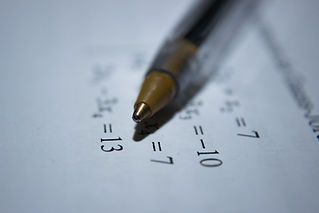top of page## Math-track

Beginning with the end in mind, when students are done with Math-track at River Tech, what should they know?

Algebra. Our curriculum is built around mastery of Algebra. We start with arithmetic (addition, subtraction, multiplication, and division), then move on to Pre-Algebra (fractions, decimals, and percentage), and then move on to Algebra (Geometry is taught in Tech-track). See our full math curriculum here.What Math curriculum does River Tech use?

Our Math curriculum utilizes a combination of: (A) teacher instruction and assistance, (B) a digital math curriculum, and (C) math workbooks. For grade level 1-5, we use Splash Math (together with textbooks).For grade level 6-12, we use School Yourself (together with textbooks).What are “Living Books”?

Living Books is a term that homeschoolers often use. It means quality literature that is well written and educational. Here are some of the living books River Tech uses in Math-track:
Adventures of Penrose the Mathematical Cat
The I Hate Mathematics! Book
Math for Smarty Pants
The Man Who Counted
G is for Googol
What’s the Point of Math?
How to be Good at Math
The World of Mathematics
How to be a Math GeniusWhen will my child start learning Algebra?

We use a competency based curriculum, so each child learns at their own pace. A child who finds math difficult may progress at a similar pace as public school, while a child who easily grasps the concepts could move considerably faster. What’s great about River Tech’s approach is, we stay with a concept for as long as it is necessary to master it, and then we move on. There is no pressure to learn slower or faster than what comes natural to each child.What is taught at each level of Math-track?

See our full math curriculum here. But here is a short summary to give you an idea.

Level 1: We focus on Arithmetic: Addition and Subtraction. Here we use Abeka Arithmetic, Mammoth Math, games, and living books.

Level 2: We focus on Arithmetic: Multiplication and Division. Here we use Mammoth Math, games, and living books.

Level 3: We focus on Pre-Algebra: Fractions, Decimals, and Percentage. Here we use Mammoth Math, Essential Math and living books. (Geometry is taught in Tech-track).

Level 4: Algebra. Here we use a variety of books, Khan Academy which is a free software, and living books. See our full math curriculum here.## Detailed Curriculum

#### Level 1

Level 1 focuses on addition & subtraction, but also covers other subjects like counting, writing and reading numbers, place value, money, graphs, measurement, time, temperature, Roman numerals, and simple fractions.

Books:
—Abeka
Arithmetik 1
—Abeka Arithmetik 2
—Mammoth Math: Add & Subtract 4

Plus living books and games.

#### Level 2

Focus: multiplication & division

Level 2 is laser focused on multiplication & division.

Books:

—Mammoth Math: Multiplication 1

—Mammoth Math: Division 1
—Mammoth Math: Multiplication 2
—Mammoth Math: Division 2

—Mammoth Math: Multiplication & Division 3

Plus living books and games.

#### Level 3

Focus: pre-algebra

Level 3 focuses on pre-algebra, but also covers and reviews other subjects like whole numbers, fractions, decimals, proportions, percents, some geometry (although most geometry will be taught in Tech-track), number theory, simple algebra, integers, charts, graphs, probability, statistics, and word problems.

Books:

—Math Mammoth: Introduction to Fractions

—Math Mammoth: Fractions 1

—Math Mammoth: Fractions 2

—Math Mammoth: Decimals 1
—Math Mammoth: Decimals 2

—Math Mammoth: Fractions & Decimals 3
—Math Mammoth: Percent
—Mastering Essential Math Skills 1
​—Mastering Essential Math Skills 2
—Mastering Essential Pre-Algebra

#### Level 4

Focus: Algebra

Level 4 is laser focused on Algebra.

Books:

—Mammoth Math: The Four Operations

—Mammoth Math: Expressions & Equations
—Mammoth Math: Linear Equations